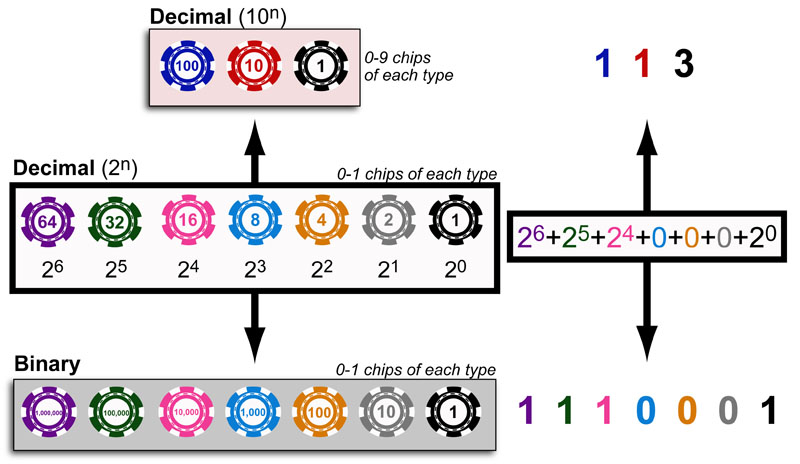# Understanding Binary with Poker ChipsComputers store information with transistors or “switches” that have an on (“1”) or off (“0”) state. As a result, they must perform mathematical and logical operations using a binary (base-2) numbering system. For example, as can be see above, computers represent our the decimal/base-10 number “113” with the binary/base-2 number: “1110001”.

These different numbering systems are different “counting-unit sets” analogous to “poker chips.” For example, for base-10 113″ you’d have 3 different types of chips/units for each power of 10: “100”, “10”, “1” and you can use 0-9 of each to count “113” = 1x“100” + 1x“10” + 3x“1”.

Similarly, for the binary number “1110001” you’d use 6 different types of chips/units for each power of 2. As such, you can convert a decimal number to a binary number by “factoring” the decimal number into the sum of several powers of 2 and then representing each power of 2 position as either a 1 or 0.